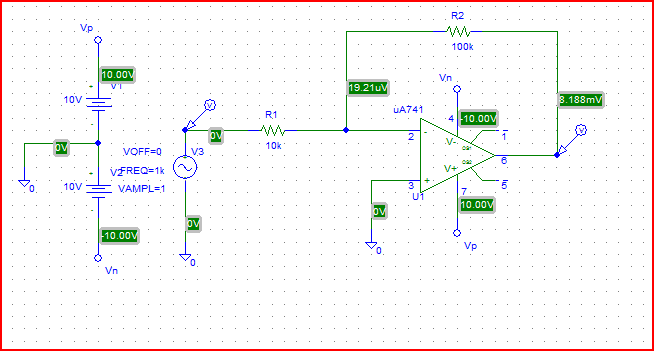# Pspice opamp DC open loop gain

## Homework Statement

Hi, im trying to use pspice student to work out a ideal opamp. This is what i did:## Homework Equations

I have find out the gain for my opamp using simulation. But the problem is how can i find out the DC open loop gain using this software? i have google it and it just show me the way of "spice" to find out the open loop gain. In my courework, i need to use the simulation.

Thanks for the help

There are a lot of ways.

The easy way is to open the loop then manually adjust the input voltage until you get a finite output voltage that's not at the rails. Then take the ratio of output to input.

This is a "C" answer. There are better ways; see if you can figure one out.

I am not quite understand what you mean by "that's not at the rails"?

The amplifier in your diagram is "closed loop" because you have a feedback resistor of 100k from the output ( terminal 6) to the - input ( terminal 2)
Open loop means removing this feedback resistor ( the open loop gain will be very, very high !!!!!!!)
"not at the rails" means the output voltage is less than the power supply voltages +/- 10V ( power supply connections are sometimes called " the rails")

Thanks for the info. I have simulate the open loop gain out but it seems like wrong.
waveform: http://www.sendspace.com/file/2mrn69
Do you see what is the problem with that?

I have connected a cap paralle with R2 to decrease the noise. And how can i find out the offset voltage for my opamp? It said i have to connect the terminal 1 and 5 to zero to get the offset voltage. But i am still confusing how can i do that?